Subsections

# Partition Function and Free Energy

Put off thy shoes from off thy feet, for the place whereon thou standest is holy ground.

Exodus, 3:5

## Definitions

The normalization constant in the canonical ensemble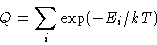is called partition function . The quantity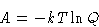is called free energy .

## Derivatives

Let us calculate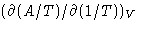: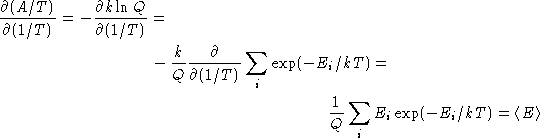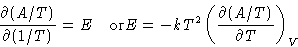If we know partition function, we can calculate average energy!

Conjugated Fields:
Variable X is a conjugated field to xi, if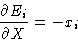If one is extensive, another is intensive!
Examples:
pressure (x) & volume (X), magnetization (x) & field (X),...
Let us calculate derivative: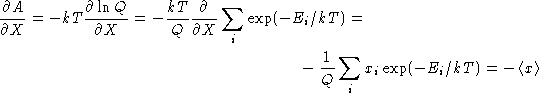If we know partition function, we can calculate averages!

Second derivative: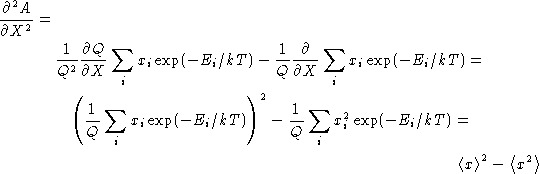This is mean squared fluctuation!

We obtained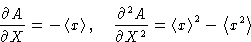(5)

We can obtain all information about our system from differentiating free energy!

© 1997 Boris Veytsman and Michael Kotelyanskii
Thu Sep 4 21:28:23 EDT 1997